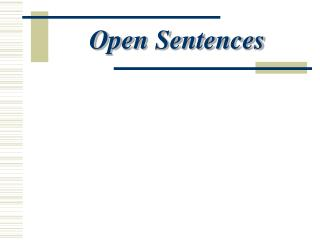DownloadDownload PresentationOpen Sentences

# Open Sentences

Télécharger la présentation## Open Sentences

- - - - - - - - - - - - - - - - - - - - - - - - - - - E N D - - - - - - - - - - - - - - - - - - - - - - - - - - -
##### Presentation Transcript

1. Open Sentences

2. Vocabulary • Open Sentence – a mathematical statement (sentence) that contains one or more variables, or unknown numbers • An open sentence is neither true nor false until the variable(s) have been replaced by intended values called replacement sets • Replacement Set – intended values or a set of numbers that are substituted into an equation to determine if they are solutions or if they satisfy the open sentence

3. Vocabulary • Solving the open sentence means finding a value from the replacement set that will make the open sentence a true statement. • A solution means the replacement number that actually makes the equation a true statement. • An open sentence may have one solution, more than one solution, or no solutions.

4. Vocabulary • Set means a collection of objects or numbers and are represented by using braces {}. • Element means each object or number in the set is called an element, or member of the set. • Sets are named by using capital letters. Examples of sets are A = {1,2,3,}; B = {6,8,10}; C= {1,2,3,6,8,10}. • The Solution set of an open sentence is the set of all replacements for the variable that will satisfy or make the equation true.

5. Vocabulary: Equation • Equation – an equation states that two expressions are equal. The expressions can be variable or numeric and are represented on each side of an equal sign. Equations are separated by an equal sign Numeric Expression Variable Expression

6. Open Sentence Example 1 State whether the equation is true or false for the given value of the variable. Separating an equation by a semi-colon and indicating the value of the variable means to substitute the number into the equation to see if it is a true solution. Substitute 3 into the equation for the variable x and solve. True when x = 3 Substitute 7 into the equation for the variable x and solve. False when x = 7

7. Open Sentence Example 2 State whether the equation is true or false for the given value of the variable. Separating an equation by a semi-colon and indicating the value of the variable means to substitute the number into the equation to see if it is a true solution. Substitute 3 into the equation for the variable x and solve. True when x = 3 Substitute 2 into the equation for the variable x and solve. False when x = 2

8. Open Sentence Example 3 Find the solution or solutions for the equation for a given SET of replacement values. Substitute each value in the replacement set for the variable x. False when x = 5 True when x = 6 False when x = 7 The solution set for x + 9 = 15 is {6}.

9. Open Sentence Example 4 Find the solution or solutions for the equation for a given SET of replacement values. Substitute each value in the replacement set for the variable x. False when x = 56 False when x = 58 False when x = 60 The solution set for x - 12 = 42 is No Solution, { } for this replacement set of {56,58,60}.

10. Open Sentence Example 5 Find the solution or solutions for the equation for the given replacement set. Substitute ALL WHOLE NUMBERS for the variable x. Try a variety of whole numbers, some sentences have one number that works, some have several that work, some have none. True when x = 5 True when x = 20 True when x = 20 The solution of the equation 2x = x + x is true for any whole number value. We say this sentence has infinite solutions.

11. Vocabulary: Inequality • Equations are separated by equal signs. • Mathematical sentences that have symbols separating each side such as <, , > or  are called inequalities. The symbols are called inequality symbols. • < means less than.  means less than or equal to. •  means greater than.  means greater than or equal to.

12. Open Sentence Example 6 Find the solution or solutions for the equation for the given replacement set. Separating an inequality by a semi-colon and indicating the value of the variable means to substitute the number(s) into the inequality to see if they are a true solution. Substitute each value in the replacement set for the variable x. True when x = 8 False when x = 9 False when x = 10 The sentence is only true when x = 8. Therefore, the solution set is {8}.

13. Open Sentence Example 7 Find the solution or solutions for the equation for the given replacement set. Substitute each value in the replacement set for the variable x. False when x = 7 True when x = 8 True when x = 9 The sentence is true when x = 8 and x = 9. The solution set is {8,9}.